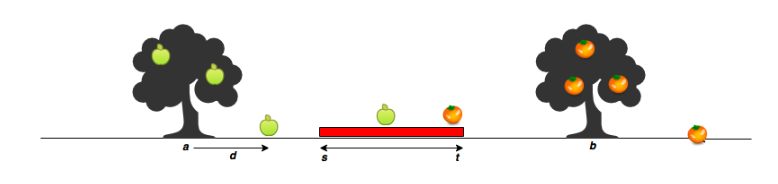# [Python] A program to find the number of apples and oranges that can be harvested

This is a personal memo.

## question

Count the number of apples that have entered the premises of the house (coordinates s ~ t).s: The starting point of the house t: End of house a: Position of apple tree b: Position of the orange tree apples: Apple fall points (relative) oranges: Orange fall point (relative)

URL

▼sample input

#### `python`

``````
s=2
t=3
a=1
b=5
apples=[-2,2,1]
oranges=[5,8,-2,-1]
``````

▼sample output

#### `python`

``````
2
1
``````

#### `python`

``````
def countApplesAndOranges(s, t, a, b, apples, oranges):
yeiledApple = 0
yeiledOrange = 0

#apple
for apple in apples:
aloc = a + apple
if s <= aloc and aloc <= t:
yeiledApple += 1

#orange
for orange in oranges:
oloc = b + orange
if s <= oloc and oloc <= t:
yeiledOrange += 1

print(yeiledApple)
print(yeiledOrange)

if __name__ == '__main__':
st = input().split()
s = int(st)
t = int(st)
ab = input().split()
a = int(ab)
b = int(ab)
mn = input().split()
m = int(mn)
n = int(mn)
apples = list(map(int, input().rstrip().split()))
oranges = list(map(int, input().rstrip().split()))

countApplesAndOranges(s, t, a, b, apples, oranges)
``````

** ・ denotes ** Represents the red region denotes his house.

**-Method output format ** print. Because it ends with method execution. countApplesAndOranges(s, t, a, b, apples, oranges)

The problem is simple, but the questions are long ...